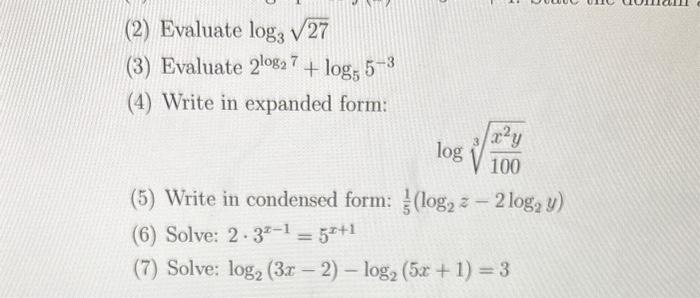# Question (2) Evaluate $$\log _{3} \sqrt{27}$$ (3) Evaluate $$2^{\log _{2} 7}+\log _{5} 5^{-3}$$ (4) Write in expanded form: $\log \sqrt{\frac{x^{2} y}{100}}$ (5) Write in condensed form: $$\frac{1}{5}\left(\log _{2} z-2 \log _{2} y\right)$$ (6) Solve: $$2 \cdot 3^{x-1}=5^{x+1}$$ (7) Solve: $$\log _{2}(3 x-2)-\log _{2}(5 x+1)=3$$Transcribed Image Text: (2) Evaluate $$\log _{3} \sqrt{27}$$ (3) Evaluate $$2^{\log _{2} 7}+\log _{5} 5^{-3}$$ (4) Write in expanded form: $\log \sqrt{\frac{x^{2} y}{100}}$ (5) Write in condensed form: $$\frac{1}{5}\left(\log _{2} z-2 \log _{2} y\right)$$ (6) Solve: $$2 \cdot 3^{x-1}=5^{x+1}$$ (7) Solve: $$\log _{2}(3 x-2)-\log _{2}(5 x+1)=3$$
Transcribed Image Text: (2) Evaluate $$\log _{3} \sqrt{27}$$ (3) Evaluate $$2^{\log _{2} 7}+\log _{5} 5^{-3}$$ (4) Write in expanded form: $\log \sqrt{\frac{x^{2} y}{100}}$ (5) Write in condensed form: $$\frac{1}{5}\left(\log _{2} z-2 \log _{2} y\right)$$ (6) Solve: $$2 \cdot 3^{x-1}=5^{x+1}$$ (7) Solve: $$\log _{2}(3 x-2)-\log _{2}(5 x+1)=3$$&#12304;General guidance&#12305;The answer provided below has been developed in a clear step by step manner.Step1/1 Solution 2) given that ,. $$\mathrm{{{\log}_{{{3}}}{\left(√{27}\right)}}}$$Write the expression in exponential form with the base of 3\( \begin{align*} &= \mathrm{{{\log}_{{{3}}}{ ... See the full answer